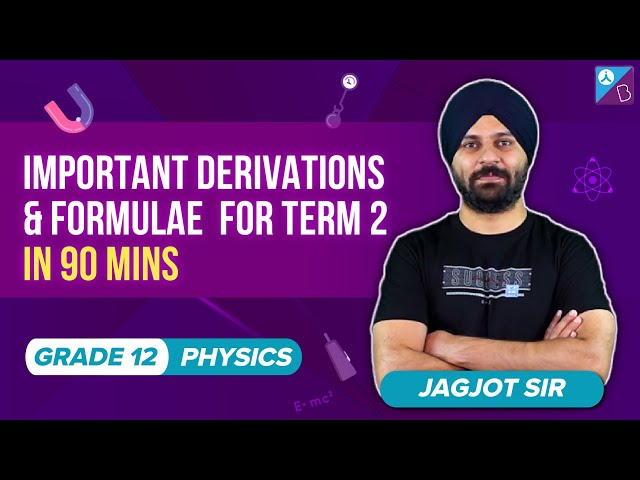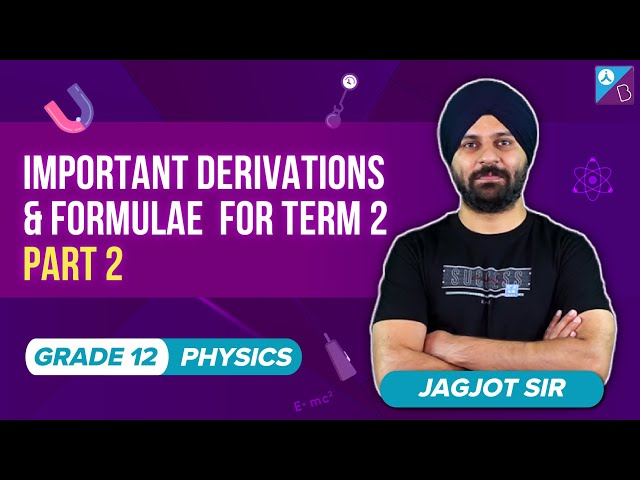Checkout JEE MAINS 2022 Question Paper Analysis : Checkout JEE MAINS 2022 Question Paper Analysis :

# IIT JEE Important Physics Formulas

Preparation of JEE (Main and Advanced) is demanding as it is among the toughest competitive exams across the globe. One of the important phases of JEE preparation is to remember all the important formulas from the entire syllabus. There will be some questions where you can directly apply the formulas and get the answers.

Therefore, such questions will be very crucial in boosting your JEE scores. Here you will learn some useful ways of memorizing formulas related to JEE Physics section. There are around 47 chapters included in JEE syllabus for Physics and remembering formulas from all these chapters is definitely a tough task.

Thus, to make things easier, we have compiled a list of important formulas for JEE Physics topics. Students can view them below.

## IIT JEE Physics Important Concept Formulas

### Steps to Memorize Formulas Effectively

There are few simple ways of memorizing formulas included in JEE Syllabus:

1. Make a handmade note of all the formulas being highlighted. This would help in proper revision and a quick glance whenever required.

2. One of the most powerful tool, not only for remembering formulas but also for solving typical Physics questions in JEE Exams is dimensional analysis. While preparing you could do a comparative study between chapters like electrostatics and magnetostatics.

For example, the energy of electric dipole is given by U = – p.E. Similarly, the energy of a magnetic dipole is U = – μ .B .

3. Additionally, remember to memorize the basic formulas like W = F.S and by using these formulas you can derive various other formulas like potential energy of a spring etc. You should memorize the values of different constants accurately because during the examination you might confuse between their values and that mistake could fetch you negative marking.

Also, Check-Out:

Stay tuned with BYJU’S to know more about JEE Mains and JEE Advanced Examination Pattern, previous years question papers, sample papers, important questions and more.

## Chapterwise Most Important Derivations and their Formulas – Physics

### Part – 1### Part – 2